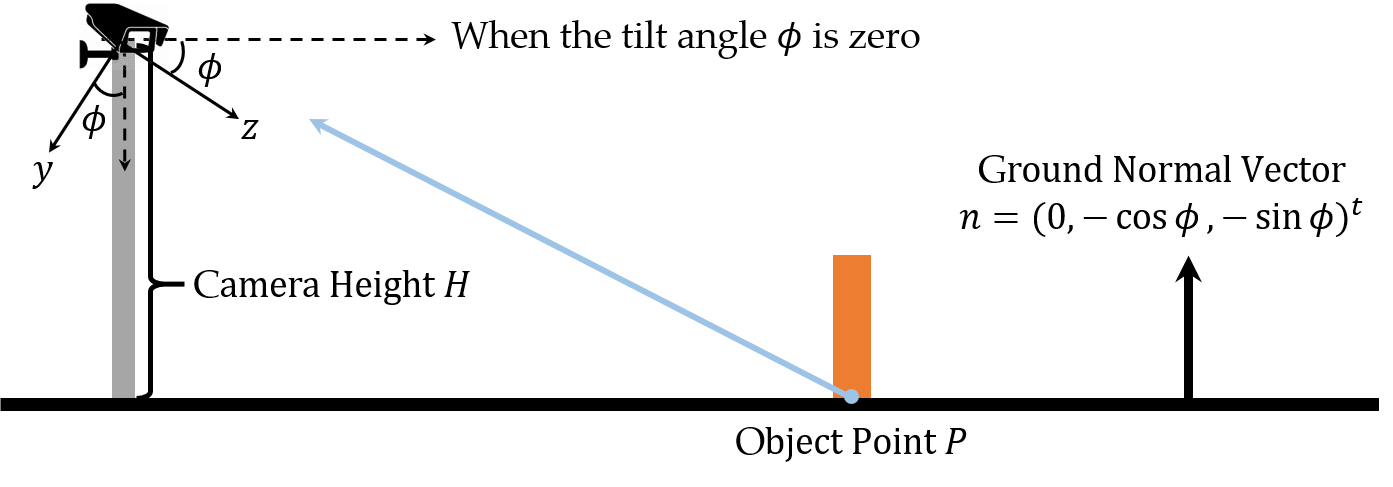# Finding Points in 3D Camera Coordinates from Points on Image PlaneWhen a camera is viewing an object on the flat ground, the bottom point $P$ of the object is projected on the camera image plane. The camera coordinate system here is right handed whose view vector is $x$-axis and $x$-axis points outwards from the screen. Let $\phi\in[0, \frac \pi 2]$ be the tilt angle of the camera, then the view vector is parallel with the ground when $\phi=0$ and intersects with a point on the ground when $\phi\not =0$. Lastly, $H$ is the camera height in meter.

## 1. The ground plane equation

If $y$-axis of the camera is rotated by $\phi$ around its $x$-axis, its $y$-axis will be parallel with the normal vector $n$ of the ground. Therefore, $-n$ is $\begin{equation} \left(\begin{array}{ccc} 1 & 0 & 0 \\ 0 & cos\phi & -sin\phi \\ 0 & sin\phi & cos\phi \end{array}\right) \left(\begin{array}{c} 0 \\ 1 \\ 0 \end{array}\right) = \left(\begin{array}{c} 0 \\ cos\phi \\ sin\phi \end{array}\right) = -n \end{equation}$

So $n$ is $(0, -cos\phi, -sin\phi)^t$. It implies that the ground plane is the plane whose normal vector is $n$ and distance from the camera origin is $H$. Therefore, the ground plane equation is $H-ycos\phi-zsin\phi=0$.

## 2. Finding $P$

$P(x, y, z)$ is the object point which is on the ground plane, so this point is satisfied with the ground plane equation. Let $P'(u, v, 1)$ be the point on the image plane and $K$ is the intrinsic matrix of the camera, then $P'$ can be written by $\begin{equation} KP = \left(\begin{array}{ccc} f & 0 & \frac w 2 \\ 0 & f & \frac h 2 \\ 0 & 0 & 1 \end{array}\right) \left(\begin{array}{c} x \\ y \\ z \end{array}\right) = \left(\begin{array}{c} fx + \frac{wz}{2} \\ fy + \frac{hz}{2} \\ z \end{array}\right) \cong \left(\begin{array}{c} \frac{fx}{z} + \frac w 2 \\ \frac{fy}{z} + \frac h 2 \\ 1 \end{array}\right) = \left(\begin{array}{c} u \\ v \\ 1 \end{array}\right) \end{equation}$

where $w$ and $h$ are the width and height of the camera. It means $P((u-\frac w 2)\frac z f, (v-\frac h 2)\frac z f, z)$, where $z$ component of $P$ is $z=\frac{H-ycos\phi}{sin\phi}(\phi\not =0)$ from the ground plane equation. Consequently, $P$ is as follows. \begin{aligned} y &= \left(v-\frac h 2\right)\frac{H-ycos\phi}{f sin\phi} \implies y = \boxed{\frac{\left(v-\frac h 2\right)H}{f sin\phi+\left(v-\frac h 2\right)cos\phi}} \\ x &= \left(u-\frac w 2\right)\frac{H-ycos\phi}{f sin\phi} \\ &= \left(u-\frac w 2\right)\frac{1}{f sin\phi} \left(H-\frac{\left(v-\frac h 2\right)Hcos\phi}{f sin\phi+\left(v-\frac h 2\right)cos\phi}\right) \\ &= \boxed{\frac{\left(u-\frac w 2\right)H}{f sin\phi+\left(v-\frac h 2\right)cos\phi}} \\ z &= \frac{1}{f sin\phi} \left(H-\frac{\left(v-\frac h 2\right)Hcos\phi}{f sin\phi+\left(v-\frac h 2\right)cos\phi}\right) = \boxed{\frac{fH}{f sin\phi+\left(v-\frac h 2\right)cos\phi}} \end{aligned}

### What if $\phi\not =0$?

When $\phi\not =0$, $z=\frac{H-ycos\phi}{sin\phi}$ is not defined because its denominator is zero. Instead, $y=H$ from the ground plane equation in this case. It also implies that $\left(v-\frac h 2 \right)\frac z f=H$ and $z=\frac{fH}{v - \frac h 2}$. Moreover, $x=\frac{\left(u-\frac w 2 \right)H}{v - \frac h 2}$ from $\left(u-\frac w 2\right)\frac z f$. Note that this result is the same with $P$ as above when $\phi=0$.

### What if $\phi\not =0$ and $v=\frac h 2$?

When $\phi\not =0$ and $v=\frac h 2$, $P$ is not defined since its all components go to infinity. But it makes sense because if the tilt angle is zero, the view vector is parallel with the ground and there is no intersection between them, or they meet at infinity.

## 3. Finding a point over $P$

Until now, $P$ is always on the ground plane. But objects are not always on the ground and they can be over the ground. In this case, it is required that the height of the object from the ground be known. Let $r$ be the height of the object, then it is possible to imagine that the ground plane is going up by $r$. It means that this point is on the new ground plane again whose distance from the camera origin is $H-r$, not $H$. Therefore, $P$ which is over the ground can be modified as follows: \begin{aligned} x &= \frac{\left(u-\frac w 2\right) \left(H-r\right)}{f sin\phi+\left(v-\frac h 2\right)cos\phi} \\ y &= \frac{\left(v-\frac w 2\right) \left(H-r\right)}{f sin\phi+\left(v-\frac h 2\right)cos\phi} \\ z &= \frac{f \left(H-r\right)}{f sin\phi+\left(v-\frac h 2\right)cos\phi} \end{aligned}

## 4. Accuracy on the image planeThe adjacent pixel points projected on the image plane from the ground may not close enough on the ground. In other words, the points that are close on the image plane can be far from each other in the camera coordinates. $\left(u_1, v_1, 1\right)^t$ and $\left(u_2, v_2, 1\right)^t$ are adjacent as above, and let $z_1$ and $z_2$ be $z$ components of the 3D points on the ground which are projected on them. \begin{aligned} z_1 &= \frac{fH}{f sin\phi+\left(v_1-\frac h 2\right)cos\phi} \implies v_1 = f \left(\frac{H}{z_1 cos\phi}-tan\phi\right) + \frac h 2 \\ z_2 &= \frac{fH}{f sin\phi+\left(v_2-\frac h 2\right)cos\phi} \implies v_2 = f \left(\frac{H}{z_2 cos\phi}-tan\phi\right) + \frac h 2 \end{aligned}

Now that $v_1$ and $v_2$ are adjacent, $v_2-v_1=1$. \begin{aligned} v_2-v_1 &= \frac{fH}{cos\phi} \left(\frac{1}{z_2} - \frac{1}{z_1} \right)=1 \implies \frac{1}{z_2} - \frac{1}{z_1} = \frac{cos\phi}{fH} \end{aligned}

This result implies that the larger $\phi$, $f$, and $H$ are, the smaller $\vert z_1-z_2 \vert$ is, the less the distance between two 3D points from $\left(u_1, v_1, 1\right)^t$ and $\left(u_2, v_2, 1\right)^t$ is.